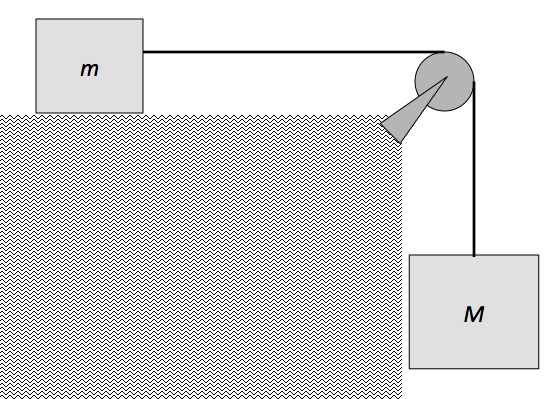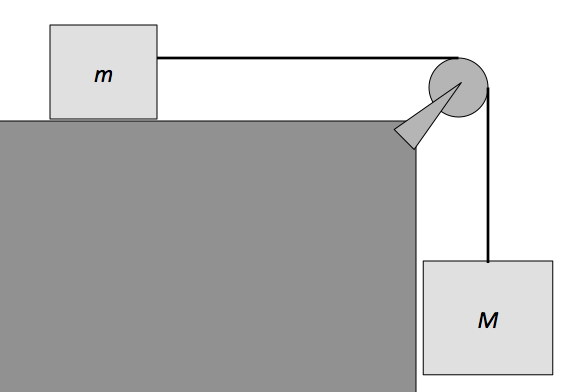Classical Mechanics

# Work 2D - Problem SolvingA mass $m = 3 \text{ kg}$ that lies on a horizontal surface is connected to another mass $M = 6 \text{ kg},$ as shown in the above diagram. The coefficient of kinetic friction between mass $m$ and the surface is $\mu_k = 0.1.$ If the system is released from rest, what is the squared velocity of mass $m ,$ when mass $M$ as descended a distance of $h = 9 \text{ m}?$

The gravitational acceleration is $g= 10 \text{ m/s}^2 .$

An object of mass $m = 8 \text{ kg}$ moves on a frictionless horizontal plane with a velocity (in meters per second) of $\vec{v} = 15 \hat{i} + 20 \hat{j}.$

If a force (in Newtons) of $\vec{F} = 4 \hat{i}$ is applied to the object for $8$ seconds, how much work does this force do to the object?

An escalator is moving downward at a constant speed of $u = 3 \text{ m/s}.$ A man of mass $m = 46 \text{ kg}$ is running upwards on it at a constant speed of $v =6 \text{ m/s}.$ If the height of the escalator is $h =2 \text{ m},$ how much work does the man do while he runs up the escalator?

A squirrel of mass $m =12 \text{ kg}$ slides up an inclined plane that makes a $\theta = 45^\circ$ angle with the horizontal, with an initial velocity of $v = 6 \text{ m/s}.$ If the coefficient of kinetic friction between the plane and the squirrel's feet is $\mu = 0.5,$ how much work does friction do to the squirrel until he comes to a stop?

The gravitational acceleration is $g= 10 \text{ m/s}^2.$A mass $m = 4 \text{ kg}$ that lies on a frictionless horizontal surface is connected to another mass $M = 5 \text{ kg}$ by a string, as shown in figure above. If the system is released from rest, what is the squared velocity of mass $m,$ when mass $M$ has descended a distance of $h = 18 \text{ m}?$

The gravitational acceleration is $g= 10 \text{ m/s}^2 .$

×